# Churn Prediction (Statistical Testing, Stacking Ensemble)# 1 Introduction¶

The objective of this notebook is to present an extensive analysis of the IBM Customer Churn Dataset and to predict the customer churn rate.

Dataset Source :

GitHub Project Repository :

NB: This project also serves as my assignments for the courses below -

You can also view this notebook on kaggle

## 1.1 Insights & Summary¶

• Dataset mostly has categorical variables
• Data is not normally distributed, performed Nonparametric Statistical tests
• Performed statistical hypothesis test to check correlation , multicollinearity
• Imbalanced dataset, did experiment with different sampling techniques(e.g stratifying, imblearn - SMOTE etc)
• Tuned Hyperparameters using Optuna
• All statistical tests were performed with a 95% confidence level (i.e., p value < 0.05)
• Performed single level Stacking Ensemble with Triple Gradient boosting algorithms

# 2 Libraries & Configurations¶

## 2.1 Import Libraries¶

In [ ]:
!pip install --upgrade scipy # to calculate Cramer's V latest version of scipy needed

Requirement already satisfied: scipy in /usr/local/lib/python3.7/dist-packages (1.4.1)
Collecting scipy
|████████████████████████████████| 38.2 MB 25 kB/s
Requirement already satisfied: numpy<1.23.0,>=1.16.5 in /usr/local/lib/python3.7/dist-packages (from scipy) (1.19.5)
Installing collected packages: scipy
Attempting uninstall: scipy
Found existing installation: scipy 1.4.1
Uninstalling scipy-1.4.1:
Successfully uninstalled scipy-1.4.1
ERROR: pip's dependency resolver does not currently take into account all the packages that are installed. This behaviour is the source of the following dependency conflicts.
albumentations 0.1.12 requires imgaug<0.2.7,>=0.2.5, but you have imgaug 0.2.9 which is incompatible.
Successfully installed scipy-1.7.2

In [ ]:
"""Import basic modules."""
import math
import os
import gc
import random
import pprint
import numpy as np               # For linear algebra
import pandas as pd              # For data manipulation
import matplotlib.pyplot as plt  # For 2D visualization
import seaborn as sns

# Warning Libraries
import warnings
warnings.filterwarnings("ignore")
# warnings.simplefilter(action='ignore', category=FutureWarning)

from collections import Counter
from scipy import stats                         # For statistics
from scipy.stats.contingency import association # upgrade scipy to use this to calculate Cramer's V

"""Plotly visualization"""
import plotly.graph_objs as go
import plotly.express as px
import plotly.io as pio
from plotly.subplots import make_subplots
from plotly.offline import init_notebook_mode, iplot

from sklearn.preprocessing import OrdinalEncoder, OneHotEncoder, LabelEncoder, StandardScaler, MinMaxScaler, RobustScaler, MaxAbsScaler
from sklearn.preprocessing import PowerTransformer # convert to Gaussian-like data
from sklearn.feature_selection import SelectKBest, f_classif, chi2

from sklearn.model_selection import StratifiedShuffleSplit, StratifiedKFold, RepeatedStratifiedKFold, train_test_split, cross_val_score
from sklearn.pipeline import Pipeline, make_pipeline

from imblearn.over_sampling import SMOTE
from imblearn.under_sampling import RandomUnderSampler

# Algorithms
from sklearn.ensemble import StackingClassifier, RandomForestClassifier, ExtraTreesClassifier
from sklearn.linear_model import LogisticRegression
from sklearn.neighbors import KNeighborsClassifier
from sklearn.svm import SVC

# Boosting Algorithms
!pip install catboost
from xgboost                          import XGBClassifier
from catboost                         import CatBoostClassifier, Pool
from lightgbm                         import LGBMClassifier

!pip install optuna
import optuna
from optuna.visualization import plot_optimization_history, plot_param_importances

import multiprocessing
import pickle, joblib
from sklearn.metrics import matthews_corrcoef, roc_auc_score, precision_recall_curve, confusion_matrix, classification_report, roc_curve, auc

from sklearn.utils import resample
from IPython.display import Markdown, display

# utility function to print markdown string
def printmd(string):
display(Markdown(string))

# customize as needed
plt_params = {
# 'figure.facecolor': 'white',
'axes.facecolor' : 'white',

## to set size
# 'legend.fontsize': 'x-large',
# 'figure.figsize': (15, 10),
# 'axes.labelsize': 'x-large',
# 'axes.titlesize': 'x-large',
# 'xtick.labelsize': 'x-large',
# 'ytick.labelsize': 'x-large'
}

plt.rcParams.update(plt_params)

sns.set_style('whitegrid')

# init_notebook_mode(connected=True)
# pio.renderers.default='notebook' # to display plotly graph
%matplotlib inline


### Check Version¶

In [ ]:
!pip freeze | grep optuna
!pip freeze | grep xgboost
!pip freeze | grep catboost
!pip freeze | grep lightgbm
!pip freeze | grep plotly
!pip freeze | grep scipy
!pip freeze | grep scikit-learn

optuna==2.10.0
xgboost==0.90
catboost==1.0.1
lightgbm==2.2.3
plotly==4.4.1
scipy==1.7.1
scikit-learn==0.22.2.post1


## 2.2 Configurations¶

In [ ]:
# padding value

# seed value
SEED = 42

# set optuna verbosity level

def seed_everything(seed=42):
random.seed(seed)
os.environ['PYTHONHASHSEED'] = str(seed)
np.random.seed(seed)

seed_everything(SEED)


# 3 Descriptive Analysis¶

Feature Name Description Data Type
customerID Contains customer ID categorical
gender whether the customer female or male categorical
SeniorCitizen Whether the customer is a senior citizen or not (1, 0) numeric, int
Partner Whether the customer has a partner or not (Yes, No) categorical
Dependents Whether the customer has dependents or not (Yes, No) categorical
tenure Number of months the customer has stayed with the company numeric, int
PhoneService Whether the customer has a phone service or not (Yes, No) categorical
MultipleLines Whether the customer has multiple lines r not (Yes, No, No phone service) categorical
InternetService Customer’s internet service provider (DSL, Fiber optic, No) categorical
OnlineSecurity Whether the customer has online security or not (Yes, No, No internet service) categorical
OnlineBackup Whether the customer has online backup or not (Yes, No, No internet service) categorical
DeviceProtection Whether the customer has device protection or not (Yes, No, No internet service) categorical
TechSupport Whether the customer has tech support or not (Yes, No, No internet service) categorical
streamingTV Whether the customer has streaming TV or not (Yes, No, No internet service) categorical
streamingMovies Whether the customer has streaming movies or not (Yes, No, No internet service) categorical
Contract The contract term of the customer (Month-to-month, One year, Two year) categorical
PaperlessBilling Whether the customer has paperless billing or not (Yes, No) categorical
PaymentMethod The customer’s payment method (Electronic check, Mailed check, Bank transfer, Credit card) categorical
MonthlyCharges The amount charged to the customer monthly   numeric , int
TotalCharges The total amount charged to the customer  object
Churn Whether the customer churned or not (Yes or No) categorical
In [ ]:
df_churn = pd.read_csv("https://raw.githubusercontent.com/IBM/telco-customer-churn-on-icp4d/master/data/Telco-Customer-Churn.csv")

Out[ ]:
customerID gender SeniorCitizen Partner Dependents tenure PhoneService MultipleLines InternetService OnlineSecurity OnlineBackup DeviceProtection TechSupport StreamingTV StreamingMovies Contract PaperlessBilling PaymentMethod MonthlyCharges TotalCharges Churn
0 7590-VHVEG Female 0 Yes No 1 No No phone service DSL No Yes No No No No Month-to-month Yes Electronic check 29.85 29.85 No
1 5575-GNVDE Male 0 No No 34 Yes No DSL Yes No Yes No No No One year No Mailed check 56.95 1889.5 No
2 3668-QPYBK Male 0 No No 2 Yes No DSL Yes Yes No No No No Month-to-month Yes Mailed check 53.85 108.15 Yes
3 7795-CFOCW Male 0 No No 45 No No phone service DSL Yes No Yes Yes No No One year No Bank transfer (automatic) 42.30 1840.75 No
4 9237-HQITU Female 0 No No 2 Yes No Fiber optic No No No No No No Month-to-month Yes Electronic check 70.70 151.65 Yes
In [ ]:
print(f"Dataset Dimension: {df_churn.shape} rows,  {df_churn.shape} columns")

Dataset Dimension: 7043 rows,  21 columns

In [ ]:
df_churn.info()

printmd("<br>**SeniorCitizen** is already converted to ineteger<br><br>**TotalCharges** should be converted to float")

<class 'pandas.core.frame.DataFrame'>
RangeIndex: 7043 entries, 0 to 7042
Data columns (total 21 columns):
#   Column            Non-Null Count  Dtype
---  ------            --------------  -----
0   customerID        7043 non-null   object
1   gender            7043 non-null   object
2   SeniorCitizen     7043 non-null   int64
3   Partner           7043 non-null   object
4   Dependents        7043 non-null   object
5   tenure            7043 non-null   int64
6   PhoneService      7043 non-null   object
7   MultipleLines     7043 non-null   object
8   InternetService   7043 non-null   object
9   OnlineSecurity    7043 non-null   object
10  OnlineBackup      7043 non-null   object
11  DeviceProtection  7043 non-null   object
12  TechSupport       7043 non-null   object
13  StreamingTV       7043 non-null   object
14  StreamingMovies   7043 non-null   object
15  Contract          7043 non-null   object
16  PaperlessBilling  7043 non-null   object
17  PaymentMethod     7043 non-null   object
18  MonthlyCharges    7043 non-null   float64
19  TotalCharges      7043 non-null   object
20  Churn             7043 non-null   object
dtypes: float64(1), int64(2), object(18)
memory usage: 1.1+ MB


SeniorCitizen is already converted to ineteger

TotalCharges should be converted to float

Drop customerID column

In [ ]:
del df_churn["customerID"]


## 3.1 Summary of Categorical Features¶

In [ ]:
df_churn.describe(include=['object']).T

Out[ ]:
count unique top freq
gender 7043 2 Male 3555
Partner 7043 2 No 3641
Dependents 7043 2 No 4933
PhoneService 7043 2 Yes 6361
MultipleLines 7043 3 No 3390
InternetService 7043 3 Fiber optic 3096
OnlineSecurity 7043 3 No 3498
OnlineBackup 7043 3 No 3088
DeviceProtection 7043 3 No 3095
TechSupport 7043 3 No 3473
StreamingTV 7043 3 No 2810
StreamingMovies 7043 3 No 2785
Contract 7043 3 Month-to-month 3875
PaperlessBilling 7043 2 Yes 4171
PaymentMethod 7043 4 Electronic check 2365
TotalCharges 7043 6531 20.2 11
Churn 7043 2 No 5174

## 3.2 Checking Duplicates¶

In [ ]:
print('Known observations: {}\nUnique observations: {}'.format(len(df_churn.index),len(df_churn.drop_duplicates().index)))

printmd("**No duplicates Found!**")

Known observations: 7043
Unique observations: 7021


No duplicates Found!

## 3.3 Unique Values¶

In [ ]:
printmd("**Unique Values By Features**")
for feature in df_churn.columns:
uniq = np.unique(df_churn[feature])


Unique Values By Features

gender                2
SeniorCitizen         2
Partner               2
Dependents            2
tenure                73
PhoneService          2
MultipleLines         3
InternetService       3
OnlineSecurity        3
OnlineBackup          3
DeviceProtection      3
TechSupport           3
StreamingTV           3
StreamingMovies       3
Contract              3
PaperlessBilling      2
PaymentMethod         4
MonthlyCharges        1585
TotalCharges          6531
Churn                 2


# 4 Data Wrangling¶

## 4.1 Missing Values¶

In [ ]:
df_churn.isna().sum()

Out[ ]:
gender              0
SeniorCitizen       0
Partner             0
Dependents          0
tenure              0
PhoneService        0
MultipleLines       0
InternetService     0
OnlineSecurity      0
OnlineBackup        0
DeviceProtection    0
TechSupport         0
StreamingTV         0
StreamingMovies     0
Contract            0
PaperlessBilling    0
PaymentMethod       0
MonthlyCharges      0
TotalCharges        0
Churn               0
dtype: int64
In [ ]:
cat_cols = set(df_churn.columns) - set(df_churn._get_numeric_data().columns)

printmd("'**isna**' is only applicable for numerical data type<br>")
printmd("Checking missing values for object data type<br><br>")

for cat in cat_cols:
print(cat.ljust(left_padding), df_churn[cat].apply(lambda x:len(x.strip()) == 0 or x.strip().lower() == 'nan').sum())

printmd("<br>TotalCharges is an object datatype, it has **11** 'nan' value")


'isna' is only applicable for numerical data type

Checking missing values for object data type

StreamingTV           0
Contract              0
TechSupport           0
TotalCharges          11
Dependents            0
PaymentMethod         0
OnlineBackup          0
MultipleLines         0
PaperlessBilling      0
Partner               0
DeviceProtection      0
InternetService       0
Churn                 0
PhoneService          0
gender                0
OnlineSecurity        0
StreamingMovies       0


TotalCharges is an object datatype, it has 11 'nan' value

### 4.1.1 Change Data Type¶

Convert TotalCharges to numeric

In [ ]:
df_churn["TotalCharges"] = pd.to_numeric(df_churn["TotalCharges"], errors = 'coerce')


### 4.1.2 Imputation¶

In [ ]:
indices_null_tc = df_churn[df_churn["TotalCharges"].isna()].index

In [ ]:
display(df_churn.iloc[indices_null_tc])

printmd("<br>**'Tenure' (months stayed at the company) is correlated with 'TotalCharges' column**")
printmd("**when 'Tenure' is 0 , 'TotalCharges' is 0 too**")

gender SeniorCitizen Partner Dependents tenure PhoneService MultipleLines InternetService OnlineSecurity OnlineBackup DeviceProtection TechSupport StreamingTV StreamingMovies Contract PaperlessBilling PaymentMethod MonthlyCharges TotalCharges Churn
488 Female 0 Yes Yes 0 No No phone service DSL Yes No Yes Yes Yes No Two year Yes Bank transfer (automatic) 52.55 NaN No
753 Male 0 No Yes 0 Yes No No No internet service No internet service No internet service No internet service No internet service No internet service Two year No Mailed check 20.25 NaN No
936 Female 0 Yes Yes 0 Yes No DSL Yes Yes Yes No Yes Yes Two year No Mailed check 80.85 NaN No
1082 Male 0 Yes Yes 0 Yes Yes No No internet service No internet service No internet service No internet service No internet service No internet service Two year No Mailed check 25.75 NaN No
1340 Female 0 Yes Yes 0 No No phone service DSL Yes Yes Yes Yes Yes No Two year No Credit card (automatic) 56.05 NaN No
3331 Male 0 Yes Yes 0 Yes No No No internet service No internet service No internet service No internet service No internet service No internet service Two year No Mailed check 19.85 NaN No
3826 Male 0 Yes Yes 0 Yes Yes No No internet service No internet service No internet service No internet service No internet service No internet service Two year No Mailed check 25.35 NaN No
4380 Female 0 Yes Yes 0 Yes No No No internet service No internet service No internet service No internet service No internet service No internet service Two year No Mailed check 20.00 NaN No
5218 Male 0 Yes Yes 0 Yes No No No internet service No internet service No internet service No internet service No internet service No internet service One year Yes Mailed check 19.70 NaN No
6670 Female 0 Yes Yes 0 Yes Yes DSL No Yes Yes Yes Yes No Two year No Mailed check 73.35 NaN No
6754 Male 0 No Yes 0 Yes Yes DSL Yes Yes No Yes No No Two year Yes Bank transfer (automatic) 61.90 NaN No

'Tenure' (months stayed at the company) is correlated with 'TotalCharges' column

when 'Tenure' is 0 , 'TotalCharges' is 0 too

In [ ]:
display(df_churn[df_churn.tenure == 1].head(2))

printmd("<br>**'TotalCharges' is the same as 'MonthlyCharges' when 'Tenure' is not 0**")

gender SeniorCitizen Partner Dependents tenure PhoneService MultipleLines InternetService OnlineSecurity OnlineBackup DeviceProtection TechSupport StreamingTV StreamingMovies Contract PaperlessBilling PaymentMethod MonthlyCharges TotalCharges Churn
0 Female 0 Yes No 1 No No phone service DSL No Yes No No No No Month-to-month Yes Electronic check 29.85 29.85 No
20 Male 1 No No 1 No No phone service DSL No No Yes No No Yes Month-to-month Yes Electronic check 39.65 39.65 Yes

'TotalCharges' is the same as 'MonthlyCharges' when 'Tenure' is not 0

Therefore, impute missing values on 'TotalCharges' column with 0

In [ ]:
display(df_churn[df_churn.tenure == 3].head(2))

printmd("<br>**'TotalCharges' increases with respect to 'MonthlyCharges' and 'Tenure'**")
printmd("<br>From the above observation we can conclude that, **'TotalCharges'  = 'MonthlyCharges' x 'Tenure' + Extra Cost**")
printmd("**Therefore, imputing missing values on 'TotalCharges' column with 0**")

gender SeniorCitizen Partner Dependents tenure PhoneService MultipleLines InternetService OnlineSecurity OnlineBackup DeviceProtection TechSupport StreamingTV StreamingMovies Contract PaperlessBilling PaymentMethod MonthlyCharges TotalCharges Churn
65 Female 0 No No 3 Yes No DSL No Yes No Yes Yes Yes Month-to-month Yes Electronic check 75.3 244.1 No
74 Female 0 No Yes 3 Yes No DSL Yes No No Yes No Yes Month-to-month Yes Bank transfer (automatic) 64.5 177.4 No

'TotalCharges' increases with respect to 'MonthlyCharges' and 'Tenure'

From the above observation we can conclude that, 'TotalCharges' = 'MonthlyCharges' x 'Tenure' + Extra Cost

Therefore, imputing missing values on 'TotalCharges' column with 0

In [ ]:
df_churn['TotalCharges'].fillna(0, inplace=True)

In [ ]:
df_churn[['tenure', 'MonthlyCharges', 'TotalCharges']].describe().T

Out[ ]:
count mean std min 25% 50% 75% max
tenure 7043.0 32.371149 24.559481 0.00 9.00 29.00 55.00 72.00
MonthlyCharges 7043.0 64.761692 30.090047 18.25 35.50 70.35 89.85 118.75
TotalCharges 7043.0 2279.734304 2266.794470 0.00 398.55 1394.55 3786.60 8684.80

## 4.2 Binning¶

There are three numerical data types which can be ranked based on their values :

• Tenure, MonthlyCharges and TotalCharges

We can bin them into three levels : high, medium and low

In [ ]:
def binning_feature(feature):
plt.hist(df_churn[feature])

# set x/y labels and plot title
plt.xlabel(f"{feature.title()}")
plt.ylabel("Count")
plt.title(f"{feature.title()} Bins")
plt.show()

bins = np.linspace(min(df_churn[feature]), max(df_churn[feature]), 4)
printmd("**Value Range**")

printmd(f"Low ({bins : .2f} - {bins: .2f})")
printmd(f"Medium ({bins: .2f} - {bins: .2f})")
printmd(f"High ({bins: .2f} - {bins: .2f})")
group_names = ['Low', 'Medium', 'High']

df_churn.insert(df_churn.shape-1,f'{feature}-binned', pd.cut(df_churn[feature], bins, labels=group_names, include_lowest=True))

# count values
printmd("<br>**Binning Distribution**<br>")
display(df_churn[f'{feature}-binned'].value_counts())

# plot the distribution of each bin
plt.bar(group_names, df_churn[f'{feature}-binned'].value_counts())

# set x/y labels and plot title
plt.xlabel(f"{feature.title()}")
plt.ylabel("Count")
plt.title(f"{feature.title()} Bins")
plt.show()


### 4.2.1 Tenure¶

In [ ]:
binning_feature('tenure')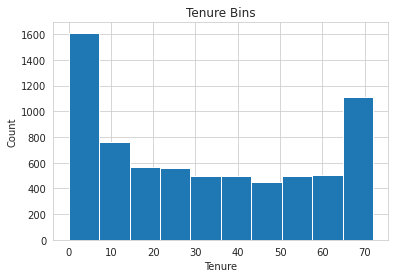Value Range

Low ( 0.00 - 24.00)

Medium ( 24.00 - 48.00)

High ( 48.00 - 72.00)

tenure tenure-binned
0 1 Low
1 34 Medium
2 2 Low
3 45 Medium
4 2 Low
5 8 Low
6 22 Low
7 10 Low
8 28 Medium
9 62 High

Binning Distribution

Low       3210
High      2239
Medium    1594
Name: tenure-binned, dtype: int64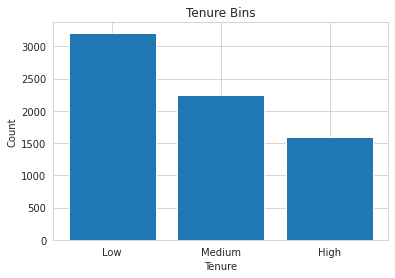### 4.2.2 MonthlyCharges¶

In [ ]:
binning_feature('MonthlyCharges')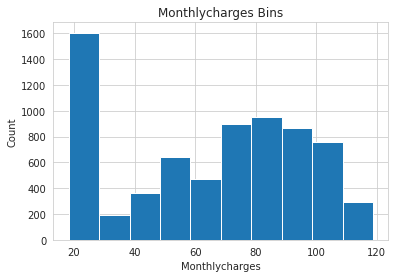Value Range

Low ( 18.25 - 51.75)

Medium ( 51.75 - 85.25)

High ( 85.25 - 118.75)

MonthlyCharges MonthlyCharges-binned
0 29.85 Low
1 56.95 Medium
2 53.85 Medium
3 42.30 Low
4 70.70 Medium
5 99.65 High
6 89.10 High
7 29.75 Low
8 104.80 High
9 56.15 Medium

Binning Distribution

Low       2451
Medium    2439
High      2153
Name: MonthlyCharges-binned, dtype: int64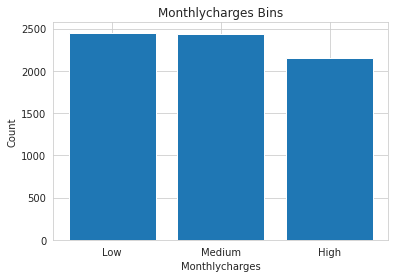### 4.2.3 TotalCharges¶

In [ ]:
binning_feature('TotalCharges')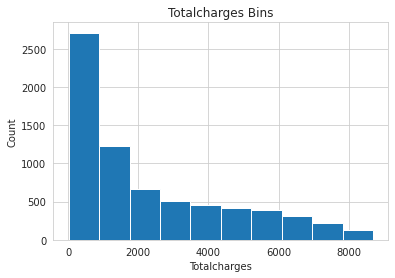Value Range

Low ( 18.80 - 2907.47)

Medium ( 2907.47 - 5796.13)

High ( 5796.13 - 8684.80)

TotalCharges TotalCharges-binned
0 29.85 Low
1 1889.50 Low
2 108.15 Low
3 1840.75 Low
4 151.65 Low
5 820.50 Low
6 1949.40 Low
7 301.90 Low
8 3046.05 Medium
9 3487.95 Medium

Binning Distribution

Low       4778
Medium    1471
High       783
Name: TotalCharges-binned, dtype: int64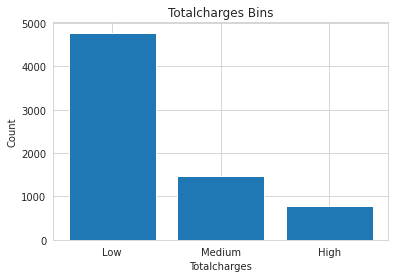Data Types Distribution after cleaning

In [ ]:
printmd("**Data Types**<br>")
df_churn.dtypes.value_counts()


Data Types

Out[ ]:
object      16
category     3
int64        2
float64      2
dtype: int64

# 5 Univariate Analysis¶

## 5.1 Statistical Normality Tests¶

Normality tests are used to determine if a dataset is normally distributed and to check how likely it is for a random variable in the dataset to be normally distributed.

Popular normality tests - D’Agostino’s K^2, Shapiro-Wilk, Anderson-Darling .

There are three numerical features in this dataset - MonthlyCharges, Tenure, and TotalCharges.

Hypotheses -

• H0: the sample has a Gaussian distribution.
• H1: the sample does not have a Gaussian distribution.

NB : we can not perform Shapiro-Wilk Test because sample size > 5000 and for this test p-value may not be accurate for N > 5000

### 5.1.1 D’Agostino’s K^2 Test¶

#### MonthlyCharges¶

In [ ]:
stat, p = stats.normaltest(df_churn['MonthlyCharges'])

print('Statistics=%.5f, p=%.3f' % (stat, p))

# interpret
alpha = 0.05
if p > alpha:
print('Sample looks Gaussian (fail to reject H0)')
else:
print('Sample does not look Gaussian (reject H0)')

Statistics=11419.52879, p=0.000
Sample does not look Gaussian (reject H0)


#### Tenure¶

In [ ]:
stat, p = stats.normaltest(df_churn['tenure'])

print('Statistics=%.5f, p=%.3f' % (stat, p))

# interpret
alpha = 0.05
if p > alpha:
print('Sample looks Gaussian (fail to reject H0)')
else:
print('Sample does not look Gaussian (reject H0)')

Statistics=76258.50517, p=0.000
Sample does not look Gaussian (reject H0)


### 5.1.2 Anderson-Darling Test¶

Hypotheses -

• H0: the sample has a Gaussian distribution
• H1: the sample does not have a Gaussian distribution

Critical values in a statistical test are a range of pre-defined significance boundaries at which the H0 can be failed to be rejected if the calculated statistic is less than the critical value. \ Rather than just a single p-value, the test returns a critical value for a range of different commonly used significance levels. \ In this case - normal/exponential (15%, 10%, 5%, 2.5%, 1%)

#### TotalCharges¶

In [ ]:
result = stats.anderson(df_churn['TotalCharges'])

print('Statistic: %.3f' % result.statistic)

p = 0

for i in range(len(result.critical_values)):
sl, cv = result.significance_level[i], result.critical_values[i]
if result.statistic < result.critical_values[i]:
print(f'Significance level {sl:.2f} % : critical value {cv:.3f}, data looks normal (fail to reject H0)')
else:
print(f'Significance level {sl:.2f} % : critical value {cv:.3f}, data does not look normal (reject H0)')

Statistic: 346.638
Significance level 15.00 % : critical value 0.576, data does not look normal (reject H0)
Significance level 10.00 % : critical value 0.656, data does not look normal (reject H0)
Significance level 5.00 % : critical value 0.787, data does not look normal (reject H0)
Significance level 2.50 % : critical value 0.917, data does not look normal (reject H0)
Significance level 1.00 % : critical value 1.091, data does not look normal (reject H0)


## 5.2 Visualization¶

### Churn (Target) Distribution¶

In [ ]:
fig = px.pie(df_churn['Churn'].value_counts().reset_index().rename(columns={'index':'Type'}), values='Churn', names='Type', title='Churn (Target) Distribution')
fig.update_traces(textposition='inside', textinfo='percent+label')
fig.show()
printmd("### Target distribution is Imbalanced")


### OnlineSecurity, OnlineBackup, DeviceProtection, TechSupport¶

In [ ]:
# Create subplots: use 'domain' type for Pie subplot
fig = make_subplots(rows=2, cols=2, specs=[[{'type':'domain'}, {'type':'domain'}],[{'type':'domain'}, {'type':'domain'}]])

1, 1)
1, 2)
2, 1)
2, 2)

# donut-like pie chart
fig.update_traces(hole=.5, hoverinfo="label+percent")

fig.update_layout(

# Add annotations in the center of the donut pies.
annotations=[dict(text='Online<br>Security', x=0.195, y=0.85, font_size=20, showarrow=False),
dict(text='Online<br>Backup', x=0.805, y=0.84, font_size=20, showarrow=False),
dict(text='Device<br>Protection', x=0.185, y=0.18, font_size=20, showarrow=False),
dict(text='Tech<br>Support', x=0.805, y=0.18, font_size=20, showarrow=False)])
fig.update_layout(margin=dict(t=0, b=0, l=0, r=0))
fig.show()

printmd("### 'Online Backup', 'Device Protection' and 'Online Security', 'Tech Support' has similar distribution")


### PaymentMethod¶

In [ ]:
display(px.pie(df_churn['PaymentMethod'].value_counts().reset_index().rename(columns={'index':'Type'}), values='PaymentMethod', names='Type', title='Payment Method Distribution'))

printmd("#### Most of the customers use E-check")


### Gender¶

In [ ]:
sns.catplot(x="gender", kind="count", data=df_churn)
plt.show()

printmd("#### Approximately 50/50 gender ratio")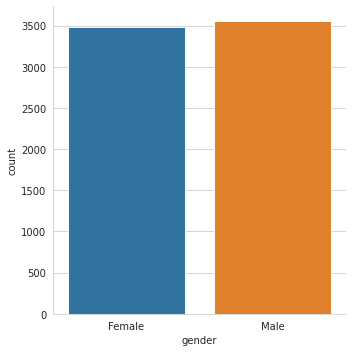### Dependents¶

In [ ]:
sns.catplot(x="Dependents", kind="count", data=df_churn)
plt.show()

printmd("#### Users who have non-dependents are approximately two times more than users having dependents")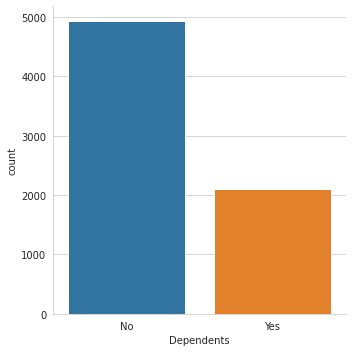### Senior Citizen¶

In [ ]:
sns.catplot(x="SeniorCitizen", kind="count", data=df_churn)
plt.show()

printmd("#### The majority of the users are not Senior Citizen")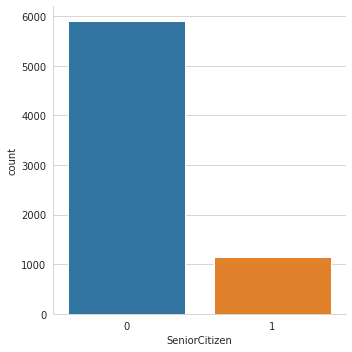### Contract¶

In [ ]:
sns.catplot(x="Contract", kind="count", data=df_churn)
plt.show()

printmd("#### Most of the users prefer Month-to-month contract")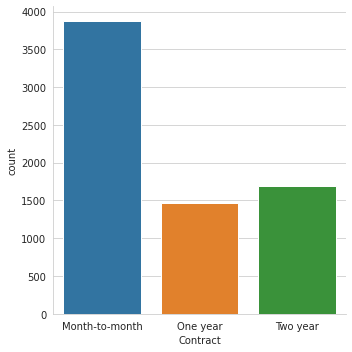### PaperlessBilling¶

In [ ]:
sns.catplot(x="PaperlessBilling", kind="count", data=df_churn)
plt.show()

printmd("#### Most of the users prefer paperless billing")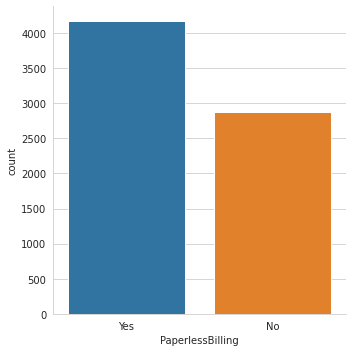### Total Charges¶

In [ ]:
sns.boxplot(x=df_churn["TotalCharges"])
plt.show()

printmd("#### The total charges fall under 4000 for majority of the users")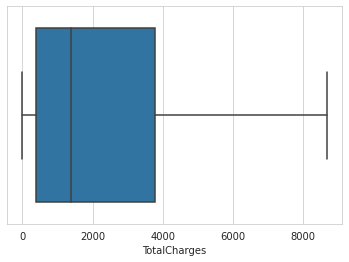### Numerical Features¶

In [ ]:
"""#1.Create a function to plot histogram and density plot."""
def plot_histogram(feature):
"""Plots histogram and density plot of a variable."""

# Create subplot object
fig = make_subplots(
rows=2,
cols=1,
print_grid=False,
subplot_titles=(f"Distribution of {feature.name} with Histogram", f"Distribution of {feature.name} with Density Plot"))

# This is a count histogram
go.Histogram(
x = feature,
hoverinfo="x+y"
),
row=1,col=1)

# This is a density histogram
go.Histogram(
x = feature,
hoverinfo="x+y",
histnorm = "density"
),
row=2,col=1)

# Update layout
fig.layout.update(
height=800,
width=870,
hovermode="closest"
)

# Update axes
fig.layout.yaxis1.update(title="<b>Abs Frequency</b>")
fig.layout.yaxis2.update(title="<b>Density(%)</b>")
fig.layout.xaxis2.update(title=f"<b>{feature.name}</b>")
return fig.show()

In [ ]:
plot_histogram(df_churn['tenure'])

printmd("**Tenure is U-shaped distributed**")


Tenure is U-shaped distributed

In [ ]:
plot_histogram(df_churn['MonthlyCharges'])

printmd("**MonthlyCharges is heavily skewed**")


MonthlyCharges is heavily skewed

In [ ]:
plot_histogram(df_churn['TotalCharges'])

printmd("**TotalCharges is reversed J-shaped distributed**")


TotalCharges is reversed J-shaped distributed

# 6 Bivariate Analysis¶

In this section, I did an extensive statistical analysis with various hypotheses testing based on paired data types like -

• numerical and numerical data
• numerical and ordinal data
• ordinal and ordinal data
• categorical and categorical data

General Hypotheses -

• H0: the two samples are independent
• H1: there is a dependency between the samples

## 6.1 List Feature Based on Types¶

In [ ]:
# Check cardinality of categorical variables
target_col_filter = df_churn.loc[:, df_churn.columns != 'Churn']

cat_cols = list(set(target_col_filter.columns) - set(target_col_filter._get_numeric_data().columns))
num_cols = list(set(target_col_filter._get_numeric_data().columns) - set({'SeniorCitizen'})) # already converted to integer

# Get number of unique entries in each column with categorical data
object_nunique = list(map(lambda col: target_col_filter[col].nunique(), cat_cols))
dict_features_by_col = dict(zip(cat_cols, object_nunique))

# Print number of unique entries by column, in ascending order
print(sorted(dict_features_by_col.items(), key=lambda x: x))

ordinal_cols = ['tenure-binned', 'MonthlyCharges-binned', 'TotalCharges-binned']
dichotomous_cols = [cat for cat in cat_cols if df_churn[cat].value_counts().count() == 2]
polytomous_cols = list(set(cat_cols) - set(dichotomous_cols) - set(ordinal_cols))


[('PhoneService', 2), ('gender', 2), ('PaperlessBilling', 2), ('Dependents', 2), ('Partner', 2), ('InternetService', 3), ('tenure-binned', 3), ('TotalCharges-binned', 3), ('MultipleLines', 3), ('Contract', 3), ('DeviceProtection', 3), ('OnlineSecurity', 3), ('OnlineBackup', 3), ('TechSupport', 3), ('StreamingMovies', 3), ('MonthlyCharges-binned', 3), ('StreamingTV', 3), ('PaymentMethod', 4)]
Categorical Columns   ['PaymentMethod', 'InternetService', 'tenure-binned', 'PhoneService', 'TotalCharges-binned', 'MultipleLines', 'Contract', 'DeviceProtection', 'gender', 'PaperlessBilling', 'OnlineSecurity', 'OnlineBackup', 'TechSupport', 'StreamingMovies', 'Dependents', 'MonthlyCharges-binned', 'Partner', 'StreamingTV']
Numerical Columns     ['MonthlyCharges', 'TotalCharges', 'tenure']
Ordinal Columns       ['tenure-binned', 'MonthlyCharges-binned', 'TotalCharges-binned']
Dichotomous Columns   ['PhoneService', 'gender', 'PaperlessBilling', 'Dependents', 'Partner']
Polytomous Columns    ['PaymentMethod', 'OnlineBackup', 'InternetService', 'TechSupport', 'StreamingMovies', 'MultipleLines', 'OnlineSecurity', 'Contract', 'DeviceProtection', 'StreamingTV']


Categorical Columns

'TechSupport', 'DeviceProtection', 'Contract', 'PaperlessBilling', 'TotalCharges-binned',
'gender', 'OnlineBackup', 'InternetService', 'StreamingTV', 'tenure-binned',
'Dependents', 'PhoneService', 'StreamingMovies', 'MultipleLines', 'Partner',
'MonthlyCharges-binned', 'OnlineSecurity', 'PaymentMethod'

Numerical Columns

'MonthlyCharges', 'tenure', 'TotalCharges'

Ordinal Columns

tenure-binned', 'MonthlyCharges-binned', 'TotalCharges-binned'

Dichotomous Columns

'PaperlessBilling', 'gender', 'Dependents', 'PhoneService', 'Partner'

Polytomous Columns

'TechSupport', 'StreamingMovies', 'DeviceProtection', 'MultipleLines', 'Contract',
'InternetService', 'OnlineSecurity', 'StreamingTV', 'PaymentMethod', 'OnlineBackup'

## 6.2 Numerical & Numerical¶

### 6.2.1 Spearman rank-order correlation¶

AKA Spearman's rho or Spearman correlation coefficient\ Applied to Continuous or ordinal

Unlike the Pearson correlation, the Spearman correlation does not assume that both datasets are normally distributed

Pearson correlation assumes normality, linearity and homoscedasticity.Pearson's correlation is also not able to tell the difference between dependent and independent variables

Hypotheses -

• H0: the two samples do not have monotonic relationship
• H1: there is a monotonic relationship between the samples

For Pearson r correlation, both variables should be normally distributed

According to the normality test tenure, MonthlyCharges and TotalCharges columns are not normally distributed

In [ ]:
def cal_spearmanr(c1, c2):

alpha = 0.05

correlation, p_value = stats.spearmanr(df_churn[c1], df_churn[c2])

print(f'{c1}, {c2} correlation : {correlation}, p : {p_value}')

if p_value > alpha:
print('Probably do not have monotonic relationship (fail to reject H0)')
else:
print('Probably have monotonic relationship (reject H0)')

In [ ]:
cal_spearmanr('tenure','MonthlyCharges')

tenure, MonthlyCharges correlation : 0.27641678933130215, p : 1.0271266876409408e-123
Probably have monotonic relationship (reject H0)

In [ ]:
cal_spearmanr('tenure','TotalCharges')

tenure, TotalCharges correlation : 0.1335958253944717, p : 2.062335511455103e-29
Probably have monotonic relationship (reject H0)

In [ ]:
cal_spearmanr('MonthlyCharges','TotalCharges')

MonthlyCharges, TotalCharges correlation : 0.2851093518219916, p : 7.880164569887811e-132
Probably have monotonic relationship (reject H0)


## 6.3 Numerical & Categorical¶

### 6.3.1 Kendall rank correlation coefficient¶

AKA Kendall's τ or Kendall's Tau

Kendall’s Tau is often used for correlation on continuous data if there are outliers in the data

A τ test is a non-parametric hypothesis test for statistical dependence based on the τ coefficient

Variable would be continuous or ordinal

Ordinal variable examples -

• age, weight, height, test scores, survey scores, yearly salary, etc
• education level (GDE/Bachelors/Masters/PhD), income level (if grouped into high/medium/low) etc

In this dataset there are three ordinal features :

• tenure-binned
• MonthlyCharges-binned
• TotalCharges-binned
In [ ]:
def kendall_rank_correlation(feature1, feature2):

coef, p_value = stats.kendalltau(df_churn[feature1], df_churn[feature2])
print(f"Correlation between {feature1} and {feature2} ")
print('Kendall correlation coefficient = %.5f, p = %.5f' % (coef, p_value))

# interpret the significance
alpha = 0.05
if p_value > alpha:
print('Samples are uncorrelated (fail to reject H0) p=%.3f' % p_value)
else:
print('Samples are correlated (reject H0) p=%.3f' % p_value)
print('----\n')

In [ ]:
ordinal_features = ['tenure-binned','MonthlyCharges-binned', 'TotalCharges-binned']

for ord in ordinal_features:
printmd(f"Correlation with **{ord}**")
kendall_rank_correlation('tenure',ord)
kendall_rank_correlation('MonthlyCharges',ord)
kendall_rank_correlation('TotalCharges',ord)


Correlation with tenure-binned

Correlation between tenure and tenure-binned
Kendall correlation coefficient = -0.28746, p = 0.00000
Samples are correlated (reject H0) p=0.000
----

Correlation between MonthlyCharges and tenure-binned
Kendall correlation coefficient = -0.10710, p = 0.00000
Samples are correlated (reject H0) p=0.000
----

Correlation between TotalCharges and tenure-binned
Kendall correlation coefficient = -0.23680, p = 0.00000
Samples are correlated (reject H0) p=0.000
----



Correlation with MonthlyCharges-binned

Correlation between tenure and MonthlyCharges-binned
Kendall correlation coefficient = -0.16485, p = 0.00000
Samples are correlated (reject H0) p=0.000
----

Correlation between MonthlyCharges and MonthlyCharges-binned
Kendall correlation coefficient = -0.22506, p = 0.00000
Samples are correlated (reject H0) p=0.000
----

Correlation between TotalCharges and MonthlyCharges-binned
Kendall correlation coefficient = -0.20858, p = 0.00000
Samples are correlated (reject H0) p=0.000
----



Correlation with TotalCharges-binned

Correlation between tenure and TotalCharges-binned
Kendall correlation coefficient = 0.07424, p = 0.00000
Samples are correlated (reject H0) p=0.000
----

Correlation between MonthlyCharges and TotalCharges-binned
Kendall correlation coefficient = 0.00298, p = 0.75152
Samples are uncorrelated (fail to reject H0) p=0.752
----

Correlation between TotalCharges and TotalCharges-binned
Kendall correlation coefficient = 0.12334, p = 0.00000
Samples are correlated (reject H0) p=0.000
----



### 6.3.2 Mann-Whitney U Test¶

The Mann-Whitney U test is a nonparametric statistical significance test for determining whether two independent samples were drawn from a population with the same distribution.

The test determines whether the medians of two or more groups are different.

NB : For the test to be effective, it requires at least 20 observations in each data sample.

• Fail to Reject H0: Sample distributions are equal. (or sample distributions are likely drawn from the same population)
• Reject H0: Sample distributions are not equal.

or

• H0: population medians are equal.
• H1: population medians are not equal.

### Correlation with Target (Dichotomous)¶

In [ ]:
def mannwhitneyu_correlation(feature1):
stat, p_value = stats.mannwhitneyu(df_churn[feature1], (df_churn['Churn'] == 'Yes').astype(int))
print(f"Correlation between {feature1} and Churn")
print('Statistics = %.5f, p = %.5f' % (stat, p_value))

# interpret the significance
alpha = 0.05
if p_value > alpha:
print('Same distribution (fail to reject H0)')
else:
print('Different distribution (reject H0)')
print('----\n')

In [ ]:
numerical_features = ['tenure','MonthlyCharges', 'TotalCharges']

for num in numerical_features:
printmd(f"Correlation with **{num}**")
mannwhitneyu_correlation(num)


Correlation with tenure

Correlation between tenure and Churn
Statistics = 48981984.50000, p = 0.00000
Different distribution (reject H0)
----



Correlation with MonthlyCharges

Correlation between MonthlyCharges and Churn
Statistics = 49603849.00000, p = 0.00000
Different distribution (reject H0)
----



Correlation with TotalCharges

Correlation between TotalCharges and Churn
Statistics = 49554833.00000, p = 0.00000
Different distribution (reject H0)
----



Biserial correlation

The point biserial correlation is used to measure the relationship between a binary variable, x, and a continuous variable, y. Like other correlation coefficients, this one varies between -1 and +1 with 0 implying no correlation. Correlations of -1 or +1 imply a determinative relationship.

NB: point-biserial correlation is conducted with the Pearson correlation formula except that one of the variables is dichotomous

The assumptions for Point-Biserial correlation include:

• Continuous and Binary
• Normally Distributed (In our case not normal)
• No Outliers
• Equal Variances

Options to normalize a non-normal distribution -

• Log transform
• Square root transform
• Box cox (can only be applied to strictly positive data)
• Yeo Johnson (both positive and negative)
In [ ]:
# https://stackoverflow.com/questions/53624804/how-to-normalize-a-non-normal-distribution

data = df_churn['MonthlyCharges'].to_numpy()
pt = PowerTransformer(method='yeo-johnson') # ‘box-cox’, 'yeo-johnson'
data = data.reshape(-1, 1)
pt.fit(data)
transformed_data = pt.transform(data)

transformed_k2, transformed_p = stats.normaltest(transformed_data)

# other methods to transform into gaussian distribution

# stats.normaltest(np.log(df_churn['MonthlyCharges']))
# stats.normaltest(np.sqrt(df_churn['MonthlyCharges'])
# stats.normaltest(stats.boxcox(df_churn['MonthlyCharges']))

# all other methods failed to convert into gaussian

alpha = 0.05
if transformed_p > alpha:
print('Sample looks Gaussian (fail to reject H0)')
else:
print('Sample does not look Gaussian (reject H0)')

Sample does not look Gaussian (reject H0)


Since the numerical columns can not be converted to gaussian distribution, point biseral correlation test can not be performed

### 6.3.3 Polytomous(Nominal) with numeric¶

For a dichotomous categorical variable and a continuous variable we can calculate a Pearson correlation if the categorical variable has a 0/1-coding for the categories. This correlation is then also known as a point-biserial correlation coefficient. (parametric test)

But when we have more than two categories for the categorical variable the Pearson correlation is not appropriate anymore. \ We should then use eta-squared, or eta, as an effect-size measure for the relationship of a categorical variable and a continuous variable.

In [ ]:
def correlation_ratio(categories, measurements):
fcat, _ = pd.factorize(categories)
cat_num = np.max(fcat)+1
y_avg_array = np.zeros(cat_num)
n_array = np.zeros(cat_num)
for i in range(0,cat_num):
cat_measures = measurements[np.argwhere(fcat == i).flatten()]
n_array[i] = len(cat_measures)
y_avg_array[i] = np.average(cat_measures)
y_total_avg = np.sum(np.multiply(y_avg_array,n_array))/np.sum(n_array)
numerator = np.sum(np.multiply(n_array,np.power(np.subtract(y_avg_array,y_total_avg),2)))
denominator = np.sum(np.power(np.subtract(measurements,y_total_avg),2))
if numerator == 0:
eta = 0.0
else:
eta = np.sqrt(numerator/denominator)
return eta

In [ ]:
correlation_ratio(df_churn['PaymentMethod'], df_churn['MonthlyCharges'])

Out[ ]:
0.40127388087245935
In [ ]:
correlation_ratio(df_churn['PaymentMethod'], df_churn['TotalCharges'])

Out[ ]:
0.35074070554475645
In [ ]:
correlation_ratio(df_churn['PaymentMethod'], df_churn['tenure'])

Out[ ]:
0.3998293691962001

## 6.4 Dichotomous & Dichotomous¶

In classification, when both of them are categorical, then the strength of the relationship between them can be measured using a Chi-square test

### 6.4.1 Phi’s correlation¶

AKA Matthews correlation coefficient (MCC)

In [ ]:
printmd("**Correlation Between Dichotomous Features with Target : Churn**")

for col in dichotomous_cols:


Correlation Between Dichotomous Features with Target : Churn

PaperlessBilling      0.1918253316664679
gender                0.0
Dependents            -0.16422140157972528
PhoneService          0.01194198002900308
Partner               -0.15044754495917656


## 6.5 Categorical & categorical¶

### 6.5.1 Chi-Square¶

detect independence between 2 categorical variables, 2x2 or 2xMany

Test statistic in the context of the chi-squared distribution with the requisite number of degrees of freedom

• If Statistic >= Critical Value: significant result, reject null hypothesis (H0), dependent.
• If Statistic < Critical Value: not significant result, fail to reject null hypothesis (H0), independent.

In terms of a p-value and a chosen significance level (alpha):

• If p-value <= alpha: significant result, reject null hypothesis (H0), dependent.
• If p-value > alpha: not significant result, fail to reject null hypothesis (H0), independent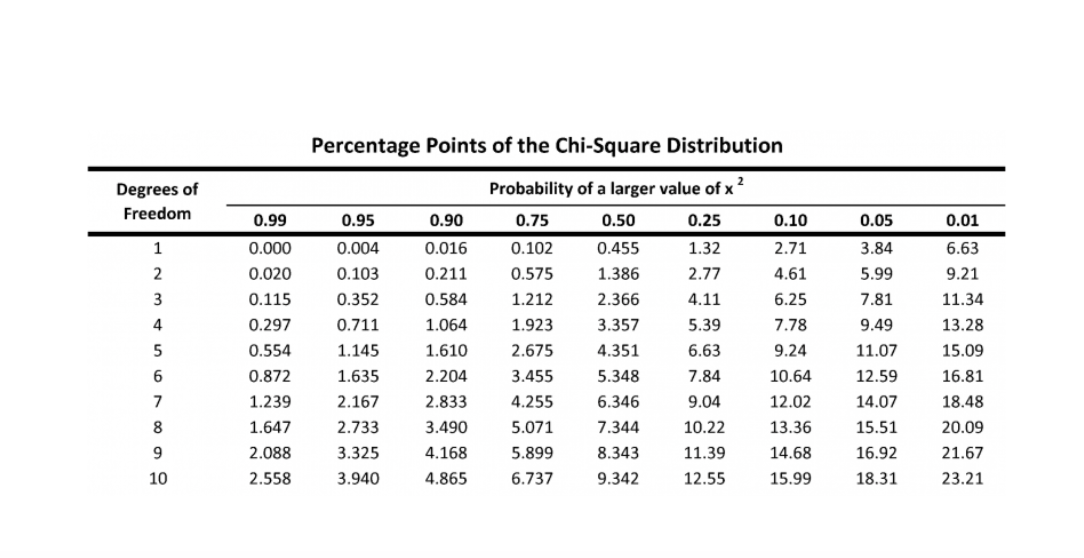In [ ]:
# alpha/significance = 0.05
# If p-value <= alpha: significant result, reject null hypothesis (H0), dependent
# If p-value > alpha: not significant result, fail to reject null hypothesis (H0), independent

def calculate_chi_square(feature1, feature2='Churn'):
printmd(f"Correlation between **{feature1}** and **{feature2}**")
crosstab = pd.crosstab(df_churn[feature1], df_churn[feature2])
# display(crosstab)
stat, p, dof, expected = stats.chi2_contingency(crosstab,correction=True)

print(f'p-value : {p}, degree of freedom: {dof}')
# print("expected frequencies :\n", expected)

# interpret test-statistic
prob = 0.95
critical = stats.chi2.ppf(prob, dof)
print('probability=%.3f, critical=%.3f, stat=%.3f' % (prob, critical, stat))

if abs(stat) >= critical:
print('Dependent (reject H0)')
else:
print('Independent (fail to reject H0)')

# interpret p-value
alpha = 1.0 - prob

print('significance=%.3f, p=%.3f' % (alpha, p))
if p <= alpha:
print('Dependent (reject H0)')
else:
print('Independent (fail to reject H0)')
print('-----------------------------------\n')

# credit : https://machinelearningmastery.com/chi-squared-test-for-machine-learning


#### Dichotomous Features¶

In [ ]:
printmd("**Chi-Squre Correlation Between Dichotomous Features with Target : Churn**")

for col in dichotomous_cols:
calculate_chi_square(col)


Chi-Squre Correlation Between Dichotomous Features with Target : Churn

Correlation between PhoneService and Churn

p-value : 0.3387825358066928, degree of freedom: 1
probability=0.950, critical=3.841, stat=0.915
Independent (fail to reject H0)
significance=0.050, p=0.339
Independent (fail to reject H0)
-----------------------------------



Correlation between gender and Churn

p-value : 0.48657873605618596, degree of freedom: 1
probability=0.950, critical=3.841, stat=0.484
Independent (fail to reject H0)
significance=0.050, p=0.487
Independent (fail to reject H0)
-----------------------------------



Correlation between PaperlessBilling and Churn

p-value : 4.073354668665985e-58, degree of freedom: 1
probability=0.950, critical=3.841, stat=258.278
Dependent (reject H0)
significance=0.050, p=0.000
Dependent (reject H0)
-----------------------------------



Correlation between Dependents and Churn

p-value : 4.9249216612154196e-43, degree of freedom: 1
probability=0.950, critical=3.841, stat=189.129
Dependent (reject H0)
significance=0.050, p=0.000
Dependent (reject H0)
-----------------------------------



Correlation between Partner and Churn

p-value : 2.1399113440759935e-36, degree of freedom: 1
probability=0.950, critical=3.841, stat=158.733
Dependent (reject H0)
significance=0.050, p=0.000
Dependent (reject H0)
-----------------------------------



With 5% significance level 'PhoneService' and 'gender' features are not dependent with the target : Churn

### Polytomous Features¶

In [ ]:
printmd("**Chi-Squre Correlation Between Polytomous Features with Target : Churn**")

for col in polytomous_cols:
calculate_chi_square(col)


Chi-Squre Correlation Between Polytomous Features with Target : Churn

Correlation between PaymentMethod and Churn

p-value : 3.6823546520097993e-140, degree of freedom: 3
probability=0.950, critical=7.815, stat=648.142
Dependent (reject H0)
significance=0.050, p=0.000
Dependent (reject H0)
-----------------------------------



Correlation between OnlineBackup and Churn

p-value : 2.0797592160864276e-131, degree of freedom: 2
probability=0.950, critical=5.991, stat=601.813
Dependent (reject H0)
significance=0.050, p=0.000
Dependent (reject H0)
-----------------------------------



Correlation between InternetService and Churn

p-value : 9.571788222840544e-160, degree of freedom: 2
probability=0.950, critical=5.991, stat=732.310
Dependent (reject H0)
significance=0.050, p=0.000
Dependent (reject H0)
-----------------------------------



Correlation between TechSupport and Churn

p-value : 1.4430840279998987e-180, degree of freedom: 2
probability=0.950, critical=5.991, stat=828.197
Dependent (reject H0)
significance=0.050, p=0.000
Dependent (reject H0)
-----------------------------------



Correlation between StreamingMovies and Churn

p-value : 2.667756755723681e-82, degree of freedom: 2
probability=0.950, critical=5.991, stat=375.661
Dependent (reject H0)
significance=0.050, p=0.000
Dependent (reject H0)
-----------------------------------



Correlation between MultipleLines and Churn

p-value : 0.0034643829548773, degree of freedom: 2
probability=0.950, critical=5.991, stat=11.330
Dependent (reject H0)
significance=0.050, p=0.003
Dependent (reject H0)
-----------------------------------



Correlation between OnlineSecurity and Churn

p-value : 2.661149635176552e-185, degree of freedom: 2
probability=0.950, critical=5.991, stat=849.999
Dependent (reject H0)
significance=0.050, p=0.000
Dependent (reject H0)
-----------------------------------



Correlation between Contract and Churn

p-value : 5.863038300673391e-258, degree of freedom: 2
probability=0.950, critical=5.991, stat=1184.597
Dependent (reject H0)
significance=0.050, p=0.000
Dependent (reject H0)
-----------------------------------



Correlation between DeviceProtection and Churn

p-value : 5.505219496457244e-122, degree of freedom: 2
probability=0.950, critical=5.991, stat=558.419
Dependent (reject H0)
significance=0.050, p=0.000
Dependent (reject H0)
-----------------------------------



Correlation between StreamingTV and Churn

p-value : 5.528994485739183e-82, degree of freedom: 2
probability=0.950, critical=5.991, stat=374.204
Dependent (reject H0)
significance=0.050, p=0.000
Dependent (reject H0)
-----------------------------------



With 5% significance level All polytomous features are dependent with the target : Churn

### 6.5.2 Cramér’s V¶

It is based on a nominal variation of Pearson’s Chi-Square Test\ Like correlation, Cramer’s V is symmetrical — it is insensitive to swapping x and y

Cramer's V is used to examine the association between two categorical variables when there is more than a 2 X 2 contingency (e.g., 2 X 3).\ In these more complicated designs, phi correlation test is not appropriate, but Cramer's statistic is. Cramer's V represents the association or correlation between two variables. This statistic is also referred to as "Cramers Phi"

In [ ]:
def cramers_v(x, y):
""" calculate Cramers V statistic for categorial-categorial association.
uses correction from Bergsma and Wicher,
Journal of the Korean Statistical Society 42 (2013): 323-328
"""
confusion_matrix = pd.crosstab(x,y)
chi2 = stats.chi2_contingency(confusion_matrix)
n = confusion_matrix.sum().sum()
phi2 = chi2/n
r,k = confusion_matrix.shape
phi2corr = max(0, phi2-((k-1)*(r-1))/(n-1))
rcorr = r-((r-1)**2)/(n-1)
kcorr = k-((k-1)**2)/(n-1)
return np.sqrt(phi2corr/min((kcorr-1),(rcorr-1)))

# credit : https://stackoverflow.com/a/46498792/11105356

In [ ]:
printmd("**Correlation Between Polytomous Features with Target : Churn**")
cramer_v_val_dict = {}
for col in polytomous_cols:
cramer_v_val_dict[col] = cramers_v(df_churn[col], df_churn['Churn'])

cramer_v_val_dict_sorted = sorted(cramer_v_val_dict.items(), key=lambda x:x, reverse=True)

for k,v in cramer_v_val_dict_sorted:

printmd("<br>**Contract, OnlineSecurity, TechSupport, InternetService are moderately correlated with Churn**<br>")


Correlation Between Polytomous Features with Target : Churn

Contract              0.40979839182553446
OnlineSecurity        0.34701606688272874
TechSupport           0.3425261587493695
InternetService       0.3220367323307425
PaymentMethod         0.3026771381187204
OnlineBackup          0.291850036724674
DeviceProtection      0.28109492388964397
StreamingMovies       0.23035147282444215
StreamingTV           0.22990176915403474
MultipleLines         0.03639958908232507


Contract, OnlineSecurity, TechSupport, InternetService are moderately correlated with Churn

In [ ]:
printmd("**Cramers V Heatmap on Polytomous Features and Target: Churn**")
cramers_v_val = pd.DataFrame(index=['Churn'], columns=polytomous_cols)

for j in range(0,len(polytomous_cols)):
u = cramers_v(df_churn['Churn'], df_churn[polytomous_cols[j]])
cramers_v_val.loc[:,polytomous_cols[j]] = u

cramers_v_val.fillna(value=np.nan,inplace=True)
plt.figure(figsize=(20,1))
sns.heatmap(cramers_v_val,annot=True,fmt='.3f', cmap="YlGnBu")
plt.show()


Cramers V Heatmap on Polytomous Features and Target: Churn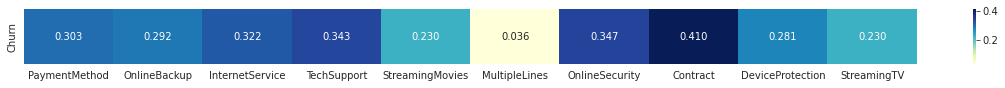#### Using Scipy Module¶

In [ ]:
crosstab = pd.crosstab(df_churn['OnlineSecurity'], df_churn['Churn'])
display(crosstab)
printmd(f"Association between OnlineSecurity and Target:Churn **{stats.contingency.association(crosstab, method='cramer')}**")

Churn No Yes
OnlineSecurity
No 2037 1461
No internet service 1413 113
Yes 1724 295

Association between OnlineSecurity and Target:Churn 0.3474004326740552

### 6.5.3 Uncertainty Coefficient¶

AKA Theil’s U - an asymmetric measure of association between categorical features

It is is based on the conditional entropy between x and y — or in human language, given the value of x, how many possible states does y have, and how often do they occur.

Formaly marked as U(x|y); Just like Cramer’s V, the output value is on the range of [0,1], where 0 means that feature y provides no information about feature x, and 1 means that feature y provides full information about features x's value

Unlike Cramer’s V, it is asymmetric

So we will not lose any valuable information unlike symmetric tests

In [ ]:
def conditional_entropy(x,y):
# entropy of x given y
y_counter = Counter(y)
xy_counter = Counter(list(zip(x,y)))
total_occurrences = sum(y_counter.values())
entropy = 0
for xy in xy_counter.keys():
p_xy = xy_counter[xy] / total_occurrences
p_y = y_counter[xy] / total_occurrences
entropy += p_xy * math.log(p_y/p_xy)
return entropy

def theil_u(x,y):
s_xy = conditional_entropy(x,y)
x_counter = Counter(x)
total_occurrences = sum(x_counter.values())
p_x = list(map(lambda n: n/total_occurrences, x_counter.values()))
s_x = stats.entropy(p_x)
if s_x == 0:
return 1
else:
return (s_x - s_xy) / s_x

In [ ]:
theilu = pd.DataFrame(index=['Churn'], columns=cat_cols)

for j in range(0,len(cat_cols)):
u = theil_u(df_churn['Churn'].tolist(),df_churn[cat_cols[j]].tolist())
theilu.loc[:,cat_cols[j]] = u

theilu.fillna(value=np.nan,inplace=True)
plt.figure(figsize=(20,1))
sns.heatmap(theilu,annot=True,fmt='.2f')
plt.show()

printmd("**Contract, OnlineSecurity, TechSupport, tenure-binned are moderately correlated with Churn**")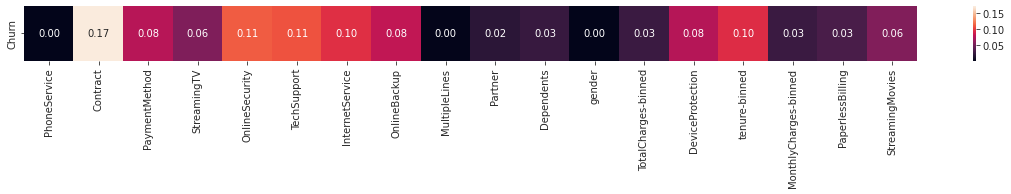Contract, OnlineSecurity, TechSupport, tenure-binned are moderately correlated with Churn

## 6.6 Collinearity¶

For categorical variables, multicollinearity can be detected with Spearman rank correlation coefficient (ordinal variables) and chi-square test (nominal variables)

For categorical and a continuous variable, multicollinearity can be measured by t-test (if the categorical variable has 2 categories, parametric) or ANOVA (more than 2 categories, parametric)

Spearman's ρ was already performed, proceeding with chi-square

### 6.6.1 Chi-Square¶

In [ ]:
calculate_chi_square('PaymentMethod','MultipleLines')


Correlation between PaymentMethod and MultipleLines


p-value : 1.1367151062832025e-81, degree of freedom: 6
probability=0.950, critical=12.592, stat=392.514
Dependent (reject H0)
significance=0.050, p=0.000
Dependent (reject H0)
-----------------------------------


In [ ]:
calculate_chi_square('PaymentMethod','PhoneService')


Correlation between PaymentMethod and PhoneService


p-value : 0.8621473788722153, degree of freedom: 3
probability=0.950, critical=7.815, stat=0.747
Independent (fail to reject H0)
significance=0.050, p=0.862
Independent (fail to reject H0)
-----------------------------------


In [ ]:
calculate_chi_square('PaymentMethod','Contract')


Correlation between PaymentMethod and Contract


p-value : 4.067638353787387e-213, degree of freedom: 6
probability=0.950, critical=12.592, stat=1001.582
Dependent (reject H0)
significance=0.050, p=0.000
Dependent (reject H0)
-----------------------------------



## 6.7 Visualization¶

### Tenure and MonthlyCharges Distribution¶

In [ ]:
plt.figure(figsize=(10,6),dpi=100)

plt.xlabel('Tenure vs Monthly Charges')
plt.ylabel('Probability Density')
plt.legend()
plt.show()

printmd("""**Both are are not normally distributed, skewed,Tenure has a
Bi-modal distribution <br>Most users stayed for less than 20 months,
Monthly Charges for most people is nearly 20 unit**""")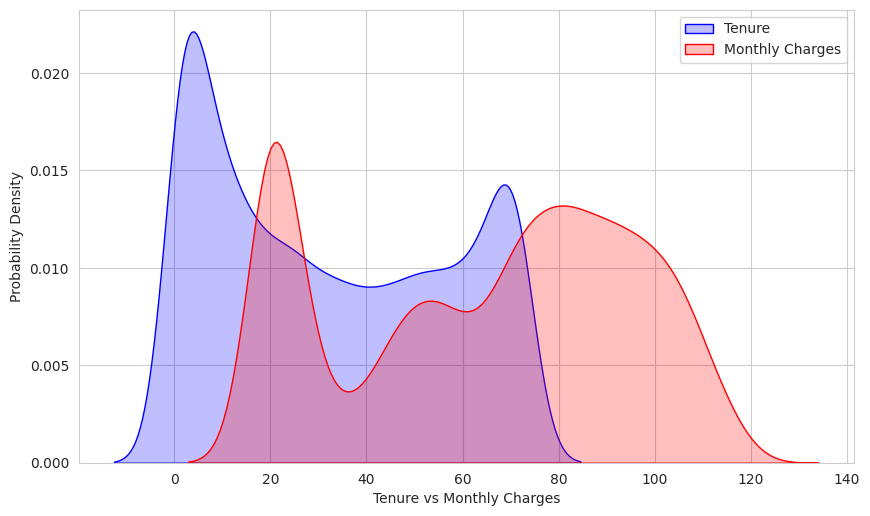Both are are not normally distributed, skewed,Tenure has a Bi-modal distribution
Most users stayed for less than 20 months, Monthly Charges for most people is nearly 20 unit

In [ ]:
# https://stackoverflow.com/a/65242391/11105356

df_g = df_churn.groupby(['StreamingTV', 'Churn']).size().reset_index()
df_g['percentage'] = df_churn.groupby(['StreamingTV', 'Churn']).size().groupby(level=0).apply(lambda x: 100 * x / float(x.sum())).values
df_g.columns = ['StreamingTV', 'Churn', 'Counts', 'Percentage']

fig = px.bar(df_g, x='StreamingTV', y='Counts',
color='Churn',
color_discrete_map={
'Yes': '#99D594',
'No': '#FC8D59',
},
text=df_g['Percentage'].apply(lambda x: '{0:1.2f}%'.format(x)))

display(fig)
printmd("**Similar ratio between streamer vs non-streamer in churned users**")